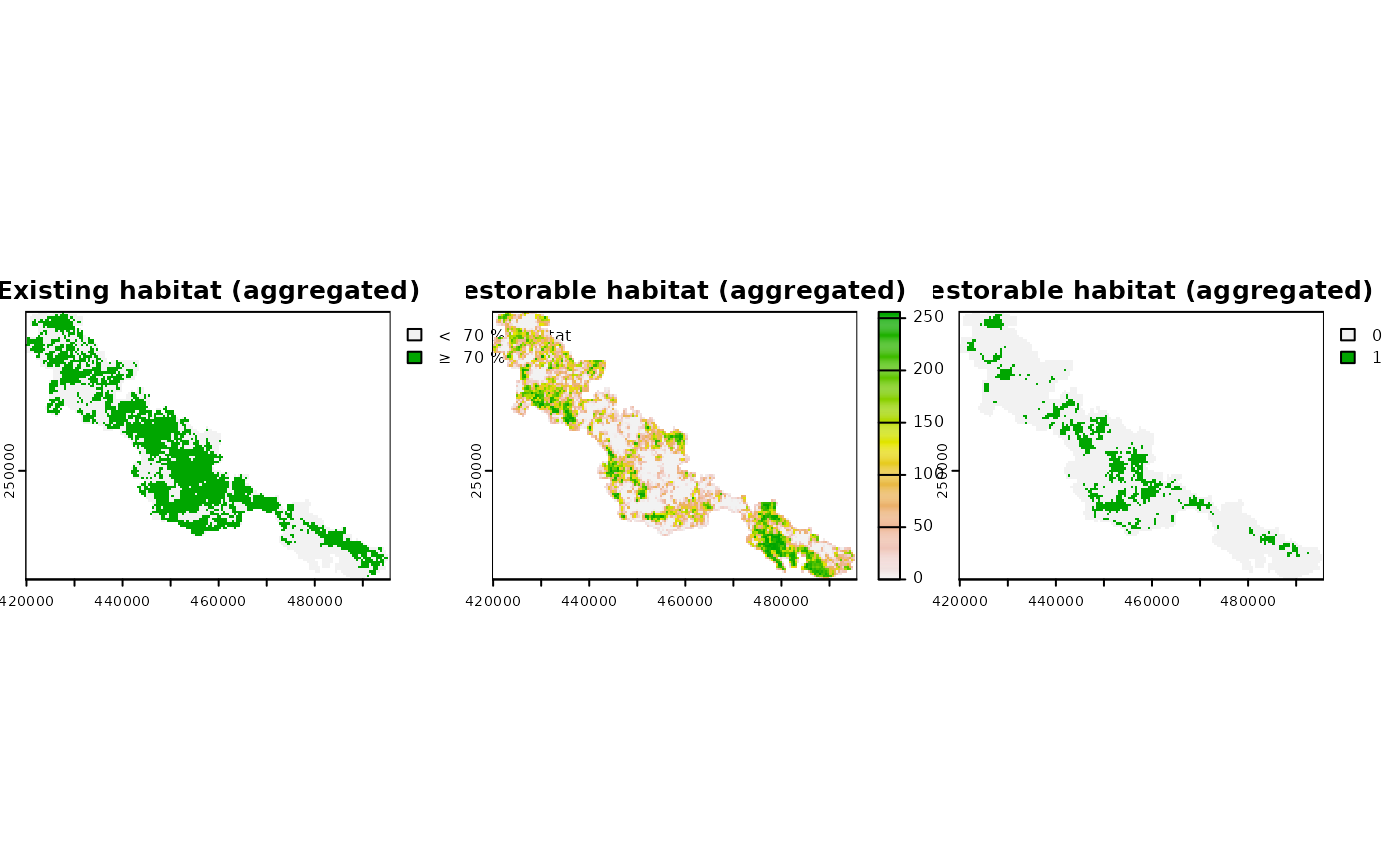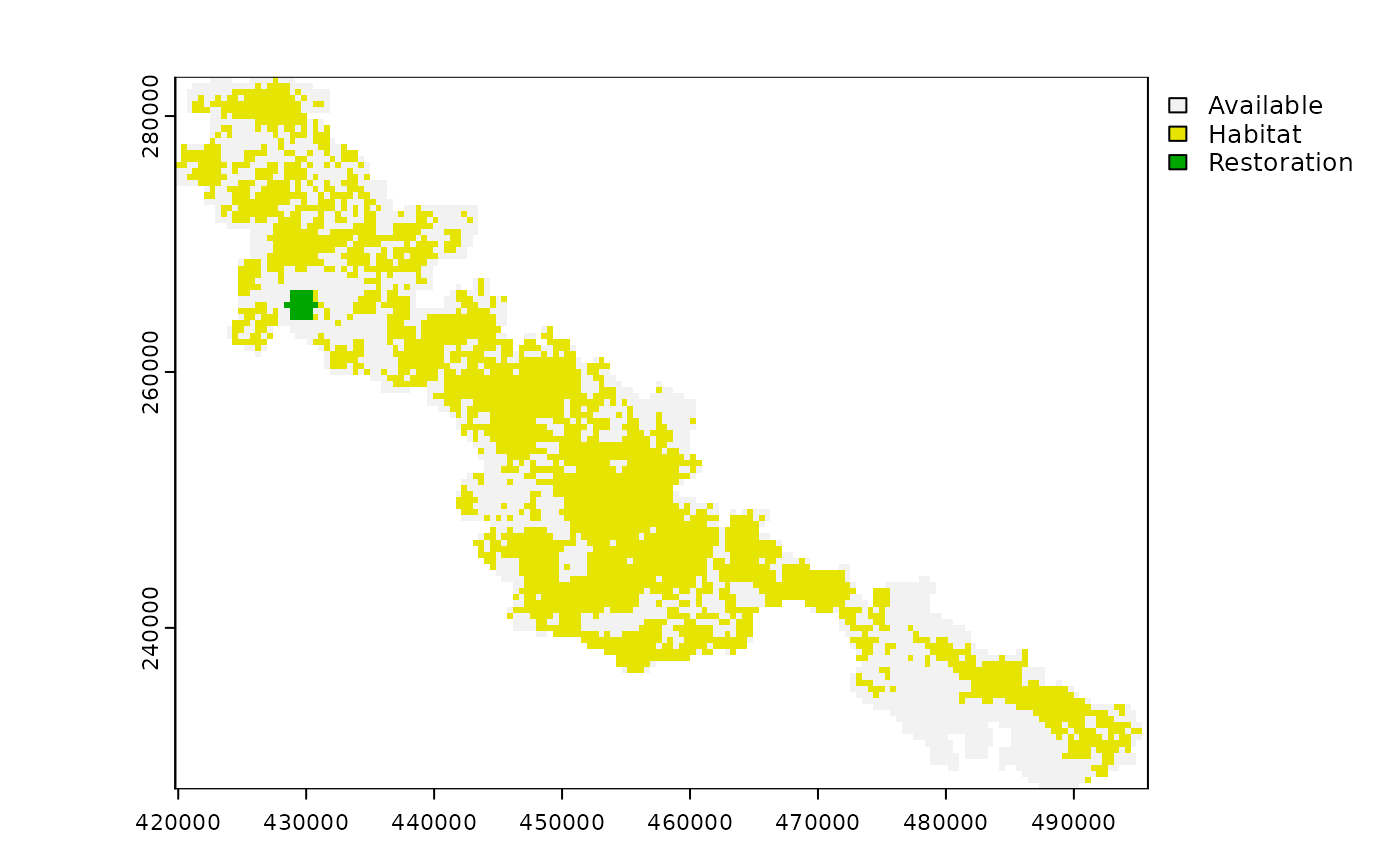Add constraint to a restoration problem (restopt_problem()) object to specify specify the available amount of surface for restoration

## Usage

add_restorable_constraint(
problem,
min_restore,
max_restore,
min_proportion = 1,
unit = "ha"
)

## Arguments

problem

restopt_problem() Restoration problem object.

min_restore

numeric Minimum allowed area to restore in the solution.

max_restore

numeric Maximum allowed area to restore in the solution

min_proportion

float Minimum habitat proportion to consider a cell as restored.

unit

unit object or a character that can be coerced to an area unit (see unit package), or "cells" for number of cells from the original habitat raster). If the input habitat raster does not use a projected coordinate system, only "cells" is available.

## Value

An updated restoration problem (restopt_problem()) object.

## Details

Given the restorable_habitat input raster in restopt_problem, this constraint ensures that the total amount of restorable habitat in selected planning units is at least min_restore and at most max_restore. The unit of min_restore and max_restore can be either in a surface unit handled by the unit package, or in number of cells from the original habitat input raster ("cells"). The min_proportion parameter is a numeric between 0 and 1, and correspond to the minimum proportion of habitat area that needs to be restored in the planning unit to consider the planning unit as restored. This proportion is relative to the area of a planning unit, which is computed automatically from the input habitat raster. Note that planning unit area is considered uniform, and the distortion is not corrected. It could be using the cellSize function of the terra package, but this function is currently pretty slow for large rasters. If your problem is at regional scale, the distortion should be negligible. However, at larger scales, the best is to use an equal-area projected coordinate system.

Note that when a solution is found, the "maximum restorable habitat" is displayed, this value does not correspond to the max_restore parameter, but to the total area that can be restored in the selected planning units. The max_restore parameter is actually an upper bound of the minimum habitat that needs to be restored to reach the min_proportion of habitat in every selected planning units.

Other constraints: add_available_areas_constraint(), add_compactness_constraint(), add_components_constraint(), add_connected_constraint(), add_locked_out_constraint(), add_min_iic_constraint(), add_min_mesh_constraint()

## Examples

# \donttest{
habitat_data <- rast(
system.file("extdata", "habitat_hi_res.tif", package = "restoptr")
)

# create problem
p <- restopt_problem(
existing_habitat = habitat_data,
aggregation_factor = 16,
habitat_threshold = 0.7
) %>%
min_restore = 200,
max_restore = 300,
min_proportion = 0.7
) %>%

# print problem
print(p)
#> -----------------------------------------------------------------
#>                          Restopt
#> -----------------------------------------------------------------
#> original habitat:     habitat_hi_res.tif
#> aggregation factor:   16
#> habitat threshold:    0.7
#> existing habitat:     in memory
#> restorable habitat:   in memory
#> -----------------------------------------------------------------
#> objective:            No optimization objective
#> -----------------------------------------------------------------
#> constraints:
#>   -  restorable (min_restore = 200, max_restore = 300, min_proportion = 0.7, unit = ha)
#>   -  compactness (max_diameter = 5, unit = cells)
#> -----------------------------------------------------------------
#> settings:
#>   - precision = 4
#>   - time_limit = 0
#>   - nb_solutions = 1
#>   - optimality_gap = 0
#>   - solution_name_prefix = Solution
#> -----------------------------------------------------------------

# plot preprocessed data
plot(rast(list(get_existing_habitat(p),
get_restorable_habitat(p),
get_locked_out_areas(p))), nc = 3)# Solve problem
s <- solve(p)
#> Good news: the solver found 1 solution satisfying the constraints ! (solving time = 0.07 s)
# plot solution
plot(s)# }